# How to calculate age in Excel: from date of birth, between two dates, and more

The tutorial shows different ways to get age from birthday in Excel. You will learn a handful of formulas to calculate age as a number of complete years, get exact age in years, months and days at today's date or a particular date.

There is no special function to calculate age in Excel, however there exist a few different ways to convert date of birth to age. This tutorial will explain the advantages and drawbacks of each way, shows how to make a perfect age calculation formula in Excel and tweak it for solving some specific tasks.

## How to calculate age from date of birth in Excel

In everyday life, the question "How old are you?" usually implies an answer indicating how many years you have been alive. In Microsoft Excel, you can make a formula to compute an exact age in months, days, hours and even minutes. But let's be traditional, and learn how to calculate age from DOB in years first.

### Basic Excel formula for age in years

How do you normally figure out someone's age? Simply by subtracting the birth date from the current date. This conventional age formula can also be used in Excel.

Assuming a birthdate is in cell B2, the formula to calculate age in years goes as follows:

`=(TODAY()-B2)/365`

The first part of the formula (TODAY()-B2) returns the difference between the current date and date of birth is days, and then you divide that number by 365 to get the numbers of years.

The formula is obvious and easy-to-remember, however, there is a tiny problem. In most cases, it returns a decimal number as demonstrated in the screenshot below.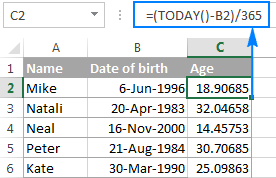To display the number of complete years, use the INT function to round a decimal down to the nearest integer:

`=INT((TODAY()-B2)/365)`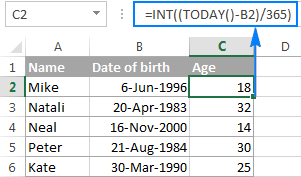Drawbacks: Using this age formula in Excel produces pretty accurate results, but not flawless. Dividing by the average number of days in a year works fine most of the time, but sometimes it gets the age wrong. For example, if someone was born on February 29 and today is February 28, the formula will make a person one day older.

As an alternative, you can divide by 365.25 instead of 365 since every fourth year has 366 days. However, this approach is not perfect either. For example, if you are calculating the age of a child who hasn't yet lived through a leap year, dividing by 365.25 produces a wrong result.

Overall, subtracting the birth date from the current date works great in normal life, but is not the ideal approach in Excel. Further on in this tutorial, you will learn a couple of special functions that calculate age faultlessly regardless of the year.

### Calculate age from date of birth with YEARFRAC function

A more reliable way to convert DOB to age in Excel is using the YEARFRAC function that returns the fraction of the year, i.e. the number of whole days between two dates.

The syntax of the YEARFRAC function is as follows:

YEARFRAC(start_date, end_date, [basis])

The first two arguments are obvious and hardly require any additional explanation. Basis is an optional argument that defines the day count basis to use.

To make a perfectly true age formula, supply the following values to the YEARFRAC function:

• Start_date - date of birth.
• End_date - TODAY() function to return the today's date.
• Basis - use basis 1 that tells Excel to divide the actual number of days per month by the actual number of days per year.

Considering the above, an Excel formula to calculate age from date of birth is as follows:

YEARFRAC(date of birth, TODAY(), 1)

Assuming the birthdate is in cell B2, the formula takes the following shape:

`=YEARFRAC(B2, TODAY(), 1)`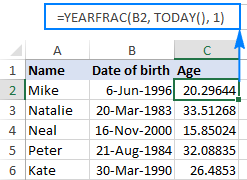Like in the previous example, the result of the YEARFRAC function is also a decimal number. To fix this, use the ROUNDDOWN function with 0 in the last argument because you don't want any decimal places.

So, here's an improved YEARFRAC formula to calculate age in Excel:

`=ROUNDDOWN(YEARFRAC(B2, TODAY(), 1), 0)`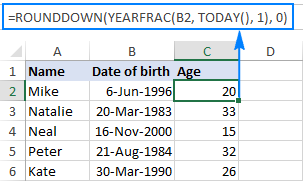### Calculate age in Excel with DATEDIF

One more way to convert date of birth to age in Excel is using the DATEDIF function:

DATEDIF(start_date, end_date, unit)

This function can return the difference between two dates in various time units such as years, months and days, depending on the value you supply in the unit argument:

• Y - returns the number of complete years between the start and end dates.
• M - returns the number of complete months between the dates.
• D - returns the number of days between the two dates.
• YM - returns months, ignoring days and years.
• MD - returns the difference in days, ignoring months and years.
• YD - returns the difference in days, ignoring years.

Since we aim to calculate age in years, we are using the "y" unit:

DATEDIF(date of birth, TODAY(), "y")

In this example, the DOB is in cell B2, and you reference this cell in your age formula:

`=DATEDIF(B2, TODAY(), "y")`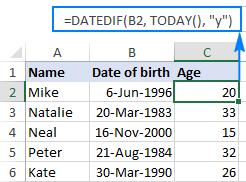No additional rounding function is needed in this case because a DATEDIF formula with the "y" unit calculates the number of full years:## How to get age from birthday in years, months and days

As you have just seen, calculating age as the number of full years that the person has lived is easy, but it is not always sufficient. If you want to know the exact age, i.e. how many years, months and days there are between someone's birth date and the current date, write 3 different DATEDIF functions:

1. To get the number of years: `=DATEDIF(B2, TODAY(), "Y")`
2. To get the number of  months: `=DATEDIF(B2, TODAY(), "YM")`
3. To get the number of days: `=DATEDIF(B2,TODAY(),"MD")`

Where B2 is the date of birth.

And then, concatenate the above functions in a single formula, like this:

`=DATEDIF(B2,TODAY(),"Y") & DATEDIF(B2,TODAY(),"YM") & DATEDIF(B2,TODAY(),"MD")`

The above formula returns 3 numbers (years, months, and days) concatenated in a single text string, as shown in the screenshot below: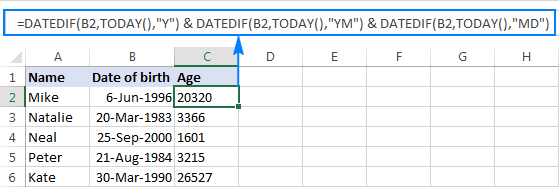Does not make much sense, uh? To make the results more meaningful, separate the numbers with commas and define what each value means:

`=DATEDIF(B2,TODAY(),"Y") & " Years, " & DATEDIF(B2,TODAY(),"YM") & " Months, " & DATEDIF(B2,TODAY(),"MD") & " Days"`

The result looks much better now: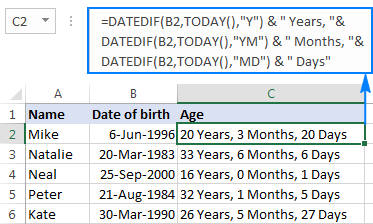The formula works great, but you can improve it even further by hiding zero values. For this, add 3 IF statements that check for 0's, one per each DATEDIF:

`=IF(DATEDIF(B2, TODAY(),"y")=0,"",DATEDIF(B2, TODAY(),"y")&" years, ")& IF(DATEDIF(B2, TODAY(),"ym")=0,"",DATEDIF(B2, TODAY(),"ym")&" months, ")& IF(DATEDIF(B2, TODAY(),"md")=0,"",DATEDIF(B2, TODAY(),"md")&" days")`

The following screenshot demonstrates the final Excel age formula in action - it returns age in years, months, and days, displaying only non-zero values: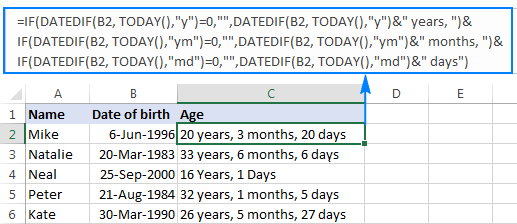Tip. If you are looking for an Excel formula to calculate age in years and months, take the above formula and remove the last IF(DATEDIF()) block that computes days.

## Specific formulas to calculate age in Excel

The generic age calculation formulas discussed above work great in most cases. In some situations, however, you may need something very specific. Of course, it's not possible to cover every and each scenario, but the following examples will give you some ideas on how you can tweak an age formula depending on your particular task.

### How to calculate age on a specific date in Excel

If you want to know someone's age at a certain date, use the DATEDIF age formula discussed above, but replace the TODAY() function in the 2nd argument with the specific date.

Assuming the birth date is in B1, the following formula will return age as of 1 January 2020:

`=DATEDIF(B1, "1/1/2020","Y") & " Years, " & DATEDIF(B1, "1/1/2020","YM") & " Months, " & DATEDIF(B1, "1/1/2020", "MD") & " Days"`

To make your age formula more flexible, you can input the date in some cell and reference that cell in your formula:

`=DATEDIF(B1, B2,"Y") & " Years, "& DATEDIF(B1,B2,"YM") & " Months, "&DATEDIF(B1,B2, "MD") & " Days"`

Where B1 is the DOB, and B2 is the date on which you want to calculate age.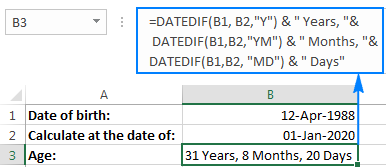### Calculate age in a certain year

This formula comes in handy in situations when the complete date to calculate at is not defined, and you know only the year.

Let's say you are working with a medical database, and your goal is to find out the patients' age at the time they underwent the last full medical examination.

Assuming the dates of birth are in column B beginning with row 3, and the year of the last medical examination is in column C, the age calculation formula goes as follows:

`=DATEDIF(B3,DATE(C3, 1, 1),"y")`

Because the exact date of the medical examination is not defined, you use the DATE function with an arbitrary date and month argument, e.g. DATE(C3, 1, 1).

The DATE function extracts the year from cell B3, makes a complete date using the month and day numbers that you supplied (1-Jan in this example), and passes that date to DATEDIF. As the result, you get the patient's age as of January 1 of a particular year: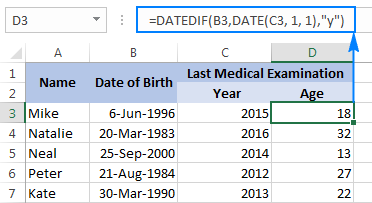### Find out a date when a person attains N years of age

Supposing your friend was born on 8 March 1978. How do you know on what date he completes his 50 years of age? Usually, you'd simply add 50 years to the person's birthdate. In Excel, you do the same using the DATE function:

`=DATE(YEAR(B2) + 50, MONTH(B2), DAY(B2))`

Where B2 is the date of birth.

Instead of hard-coding the number of years in the formula, you can reference a certain cell where your users can input any number of years (F1 in the screenshot below):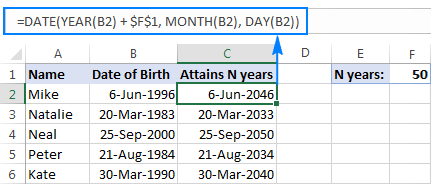### Calculate age from day, month and year in different cells

When a birthdate is split into 3 different cells (e.g. year is in B3, month in C3 and day in D3), you can calculate age in this way:

• Get the date of birth by using the DATE and DATEVALUE functions:
`DATE(B3,MONTH(DATEVALUE(C3&"1")),D3)`
• Embed the above formula into DATEDIF to calculate age from date of birth in years, months, and days:

`=DATEDIF(DATE(B3,MONTH(DATEVALUE(C3&"1")),D3),TODAY(),"y") & " Years, "& DATEDIF(DATE(B3,MONTH(DATEVALUE(C3&"1")),D3),TODAY(),"ym") & " Months, "&  DATEDIF(DATE(B3,MONTH(DATEVALUE(C3&"1")),D3),TODAY(),"md") & " Days"`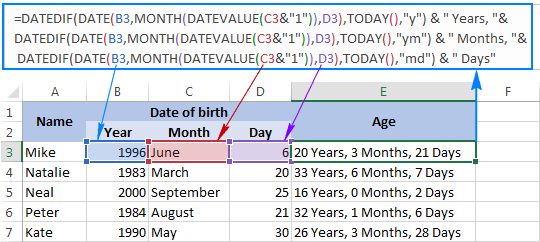## Age calculator in Excel

If you'd like to have your own age calculator in Excel, you can make one by using a few different DATEDIF formulas explained below. If you'd rather not reinvent the wheel, you can use the age calculator created by our Excel professionals.

### How to create an age calculator in Excel

Now that you know how to make an age formula in Excel, you can build a custom age calculator, for example this one:

What you see above is an embedded Excel Online sheet, so feel free to enter your birthdate in the corresponding cell, and you will get your age in a moment.

The calculator uses the following formulas to compute age based on the date of birth in cell A3 and today's date.

• Formula in B5 calculates age in years, months, and days:

`=DATEDIF(B2,TODAY(),"Y") & " Years, " & DATEDIF(B2,TODAY(),"YM") & " Months, " & DATEDIF(B2,TODAY(),"MD") & " Days"`

• Formula in B6 calculates age in months:

`=DATEDIF(\$B\$3,TODAY(),"m")`

• Formula in B7 calculates age in days:

`=DATEDIF(\$B\$3,TODAY(),"d")`

If you have some experience with Excel Form controls, you can add an option to compute age at a specific date, like shown in the following screenshot: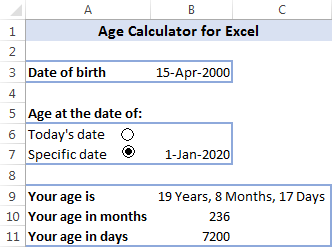For this, add a couple of option buttons (Developer tab > Insert > Form controls > Option Button), and link them to some cell. And then, write an IF/DATEDIF formula to get age either at today's date or at the date specified by the user.

The formula works with the following logic:

• If the Today's date option box is selected, value 1 appears in the linked cell (I5 in this example), and the age formula calculates based on the today date:

`IF(\$I\$5=1, DATEDIF(\$B\$3,TODAY(),"Y") & " Years, " & DATEDIF(\$B\$3,TODAY(), "YM") & " Months, " & DATEDIF(\$B\$3, TODAY(), "MD") & " Days")`

• If the Specific date option button is selected AND a date is entered in cell B7, age is calculated at the specified date:

`IF(ISNUMBER(\$B\$7), DATEDIF(\$B\$3, \$B\$7,"Y") & " Years, " & DATEDIF(\$B\$3, \$B\$7,"YM") & " Months, " & DATEDIF(\$B\$3, \$B\$7,"MD") & " Days", ""))`

Finally, nest the above functions into each other, and you will get the complete age calculation formula (in B9):
`=IF(\$I\$5=1, DATEDIF(\$B\$3, TODAY(), "Y") & " Years, " & DATEDIF(\$B\$3, TODAY(), "YM") & " Months, " & DATEDIF(\$B\$3, TODAY(), "MD") & " Days", IF(ISNUMBER(\$B\$7), DATEDIF(\$B\$3, \$B\$7,"Y") & " Years, " & DATEDIF(\$B\$3, \$B\$7,"YM") & " Months, " & DATEDIF(\$B\$3, \$B\$7,"MD") & " Days", ""))`

The formulas in B10 and B11 work with the same logic. Of course, they are much simpler because they include just one DATEDIF function to return age as the number of complete months or days, respectively.

To learn the details, I invite you to download this Excel Age Calculator and investigate the formulas in cells B9:B11.

### Ready-to-use age calculator for Excel

They users of our Ultimate Suite for Excel don't have to bother about making their own age calculator in Excel - it is only a couple of clicks away:

1. Select a cell where you want to insert an age formula, go to the Ablebits Tools tab > Date & Time group, and click the Date & Time Wizard button.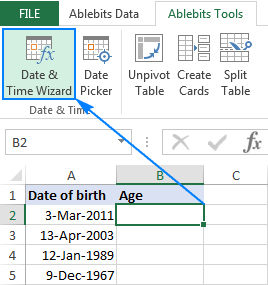2. The Date & Time Wizard will start, and you go directly to the Age tab.
3. On the Age tab, there are 3 things for you to specify:
• Data of birth as a cell reference or a date in the mm/dd/yyyy format.
• Age at today's date or specific date.
• Choose whether to calculate age in days, months, years, or exact age.
4. Click the Insert formula button.

Done!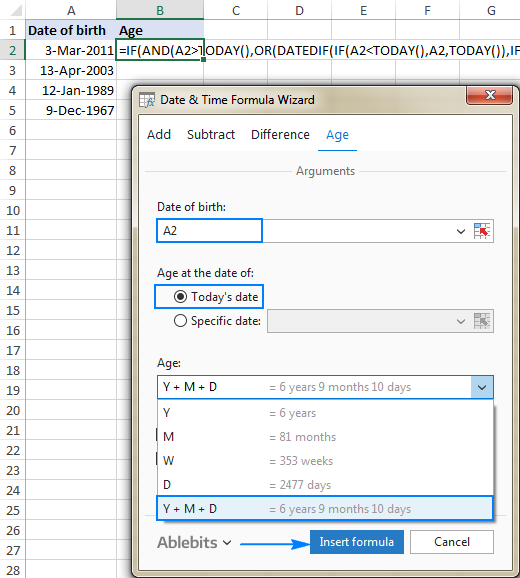The formula is inserted in the selected cell momentarily, and you double-click the fill handle to copy it down the column.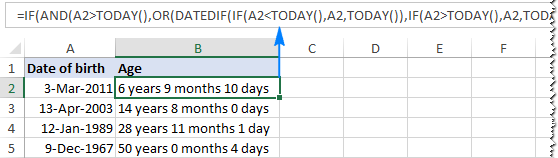As you may have noticed, the formula created by our Excel age calculator is more complex than the ones we've discussed so far, but it caters for singular and plural of time units such as "day" and "days".

If you'd like to get rid of zero units like "0 days", select the Do not show zero units check box: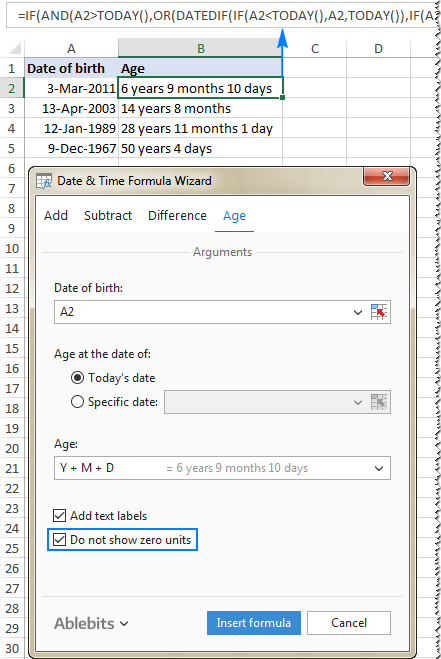If you are curious to test this age calculator as well as to discover 60 more time-saving add-ins for Excel, you are welcome to download a trial version of our Ultimate Suite. If you like the tools and decide to get a license, be sure to grab a 15% off coupon code that we provide to our valuable blog readers: AB14-BlogSpo

## How to highlight certain ages (under or over a specific age)

In some situations, you may need not only calculate age in Excel, but also highlight cells which contain ages that are under or over a particular age.

If your age calculation formula returns the number of complete years, then you can create a regular conditional formatting rule based on a simple formula like these ones:

• To highlight ages equal to or greater than 18: =\$C2>=18
• To highlight ages under 18: =\$C2<18

Where C2 is the top-most cell in the Age column (not including the column header).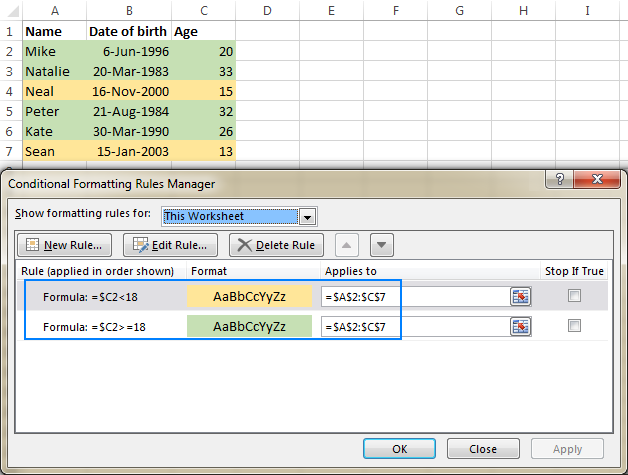But what if your formula displays age in years and months, or in years, months and days? In this case, you will have to create a rule based on a DATEDIF formula that calculates age from date of birth in years.

Supposing the birthdates are in column B beginning with row 2, the formulas are as follows:

• To highlight ages under 18 (yellow):

`=DATEDIF(\$B2, TODAY(),"Y")<18`

• To highlight ages between 18 and 65 (green):

`=AND(DATEDIF(\$B2, TODAY(),"Y")>=18, DATEDIF(\$B2, TODAY(),"Y")<=65)`

• To highlight ages over 65 (blue):

`=DATEDIF(\$B2, TODAY(),"Y")>65`

To create rules based on the above formulas, select the cells or entire rows that you want to highlight, go to the Home tab > Styles group, and click Conditional Formatting > New Rule… > Use a formula to determine which cells to format.

The detailed steps can be found here: How to make a conditional formatting rule based on formula.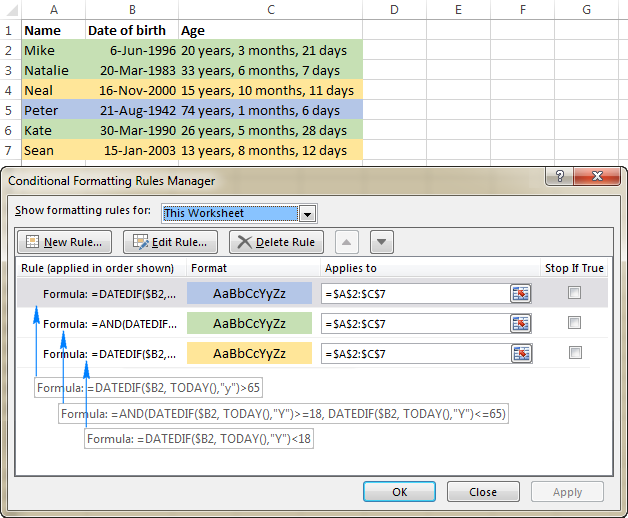This is how you calculate age in Excel. I hope the formulas were easy for you to learn and you will give them a try in your worksheets. The sample Excel Age Calculation worksheet will make things even easier. I thank you for reading and hope to see you on our blog next week!

### 56 Responses to "How to calculate age in Excel: from date of birth, between two dates, and more"

1.Kim says:

Hi,

IF(ISNUMBER(D3),1*C\$3, "0")+IF(ISNUMBER(D4),1*C\$4, "0")+IF(ISNUMBER(D5),1*C\$5, "0") . . .

•Kim says:

I'm using Microsoft Excel 2016 btw

•Svetlana Cheusheva (Ablebits.com Team) says:

Hello Kim,

There is no need to write a formula for each cell. Just write it for the topmost cell (row 3 in your case), and then drag it down to copy the formula to other cells:
=IF(ISNUMBER(D3), 1*C3, 0)

Since you use relative cell references (without the \$ sign), Excel will properly adjust the formula for each cell where it is copied.

•Kim says:

2.MR Khan says:

Dear Madam, It's awesome formula thanks for share with us.

3.Brian says:

May I offer the following example of the power of DATEDIF, but also its flaw. In my line of work I have offered circa 25,000 personnel Contract extensions in a set year sequence capped at a specific age. I examined, for example, their Hire Date first using Named Ranges in the following formula: DATEDIF(DOB,HireDate,"y")&" yrs "&DATEDIF(DOB,HireDate,"ym")&" mths "&DATEDIF(DOB,HireDate,"md")&" days"; noting that the TEXT answer would be ordered alphabetical A-Z i.e. 1, 10, 11, 12...2, 20, 21 etc. and not 1, 2, 3...I get around this TEXT ordering requirement by generating another column value that roughly divides Excel date difference by 365.25 and rounds to a suitable value. I then use a complex Nested IF statement to offer, for example, a xx year Contract (which is xx years minus 1 day) capped at xx age. It works flawlessly.

4.Slavko says:

Hello Svetlana,
I do not see the function DATEDIF the drop-down menu excel 2016. Is it because of the free student version or wrong. Sorry if something is incorrectly written. I used a translator.
My language is Serbian :)

•Svetlana Cheusheva (Ablebits.com Team) says:

Hello Slavko,

DATEDIF is one of the very few undocumented functions, and therefore it does not appear in the list of formulas (nor in the formula bar when you start typing the formula name) in any Excel version. You have to remember its syntax and type all the arguments manually.

5.karthik says:

Hi, Good Day, I have an interesting assignment given to me. I need to find out numbers between the range 3140 to 4140 whose sum should be 21. This is required for vehicle registration. Can you help me with the formula (Indirect function) in excel to my email ID, thanks.

•Renat Tlebaldziyeu (Ablebits.com Team) says:

Hi karthik,

Please try to do the following:
1. Fill the values 3140-4140 in range A1:A1001.
2. Fill the following formula in range B1:B1001:
=IF(SUMPRODUCT(VALUE(MID(A1, ROW(INDIRECT("1:"&LEN(A1))),1)))=21, "YES", "")
3. The values that meet your condition are marked with YES in column B.
4. Use the Excel Filter or the Sort feature to copy the results.

6.Romi Bagga says:

You are a pro!! :)

7.Prateek says:

Awesome !!!

8.C. Gaith says:

Amazing blog. I must say all informations are very helpful for all students and all employees. Good work. Thanks for sharing with us.

9.ali says:

My database only capture year of birth (not day, month, year). When I tried this formula it doesn't work. Any suggestions on how I can use only year of birth to determine age with a formula?
Help

•Svetlana Cheusheva (Ablebits.com Team) says:

Hi Ali,

Assuming the year of birth is in cell A2, you can use this formula:
=YEAR(TODAY())-A2

10.Rene S. says:

This is great material Svetlana, I have a question though.
Working in an international environment I have tried to share date functions with references to dates using mm dd yy etc. both in formulas for comparison reasons as well as in formulas for formatting reasons.
When I write formulas in an english based sheet which I share with colleagues in France however it does not recognize the YY for example because in the French excel version another symbol is used for Year.
Is there a way to solve this and does excel have similar issues with other country languages to your knowledge?

•Svetlana Cheusheva (Ablebits.com Team) says:

Hi Rene,

The time unit codes are indeed language-specific, therefore Excel does have similar issues with other languages. For example, German users will need to use "t" instead of "d" ("day" in German is "tag"), "j" instead of "y" ("year" is "jahr"), "m" is fine for "month" because in German it also begins with "m" (monat).

As far as I know there is no way to "fix" this other than installing the English locale of Office.

11.Cda Yusuf says:

I'm a teacher from Malaysia. A loootttttt of thank you for this tutorial.

12.RAJENDRA KUMAR says:

Very very.............. thank's for creating this formula

13.Jay says:

Hi,

I have this problem where I need to get the age from different dates.
Start date = 3/29/2017 11:47:29 AM
Stop date = 2017-03-29 12:46:10
Close date = 2017-03-31 15:40:10

I need to get the count of days if there is no close date yet, but I need to skip the stop date if there is a close date.

This formula works if there is no close date:
=IF(I71="",IFERROR(DATEDIF(C71,H71,"md"),I71)&" day/s")
I get "FALSE" when close date is populated.

Thank you,
Jay C.

•Natalia Sharashova (Ablebits.com Team) says:

Hi, Jay,
when the close date is populated, you need to use the second argument in IFERROR. You can try the formula below:

=IF(B14="",IFERROR(DATEDIF(B12,B13,"md")&"days",""),B14)

Feel free to read more about IFERROR function to understand its syntax and avoid the mistake in the future.

14.Ashu Choudhary says:

Hi,
I have calculated my Age. It is in years, months and then in days. Now I want more to calculate in hours, minute and seconds so that it would be 18 years 11 months 13 days 2 hours 56 minute 33seconds etc. If there's Any trick to find it out then Please tell us.

15.JamSmith says:

This blog is really helpful!!! It is always difficult and confusing to find out the actual age in days, months and sometimes in years. Your technique is very useful for the students and the bank employees but as you concern most of the people try to find out online tools to calculate their age in different perspectives. As I'm also using the binarytranslator tools to calculate anyone's age. And now I came across your blog so now I can calculate the age using your technique as well!!! Thanks for sharing.

16.charan says:

Hi need help in getting date of birth from age using current date.

17.Mark Smith says:

These formulas do not seem to work in Excel 2011 for the Mac

18.sarah lucken says:

Hi,

I was wondering if you could help me i am trying get children's age in months between different date throughout the year.

The children date of birth is on sheet 1 (called Info) in cell C3
The first set of dates i need it to calculate between are in cells G3 and G4 on sheet 2 (called Child A) the information needs to be calculated in months if possible 34 months - 35 months

I look forward to your help :-)

•Gennady Terekhov (Ablebits.com Team) says:

Hello, sarah lucken,

=IF(G4>G3, MONTH(G4-G3), MONTH(G3-G4))

19.taufeq says:

thank you for the explaination.

i have one question. im working on a database with children data in it (less than one year old). using this formula will make their age 100+.. is there any modification to the formula for the above problem?

thank you

•taufeq says:

the data includes older people too

20.Tim says:

Hi

Is it possible to create age calculator with Excel, what give answer like this; 45 years, 3 months, 12 days, 3 hours, 34 minutes, 15 seconds?
Birthdate is cell a1 (dd.mm.yyyy hh:mm:ss), todays-date is cell b1 (dd.mm.yyyy hh:mm:ss). Answer cell:s like this; Years is cell c1, months is cell d1, days is cell e1 and so on.. I used many many hours to figure, how to do this, but no success. Please help me.

•Gennady Terekhov (Ablebits.com Team) says:

Hello, Tim,

=DATEDIF(A1, IF(TIME(HOUR(A1), MINUTE(A1), SECOND(A1))>TIME(HOUR(B1), MINUTE(B1), SECOND(B1)),B1-1,B1),"Y")&" years"

=DATEDIF(A1, IF(TIME(HOUR(A1), MINUTE(A1), SECOND(A1))>TIME(HOUR(B1), MINUTE(B1), SECOND(B1)),B1-1,B1),"YM")&" months"

=DATEDIF(A1, IF(TIME(HOUR(A1), MINUTE(A1), SECOND(A1))>TIME(HOUR(B1), MINUTE(B1), SECOND(B1)),B1-1,B1),"MD")&" days"

=HOUR((1-VALUE(TIME(HOUR(A1), MINUTE(A1), SECOND(A1))))+VALUE(TIME(HOUR(B1), MINUTE(B1), SECOND(B1))))&" hours"

=MINUTE((1-VALUE(TIME(HOUR(A1), MINUTE(A1), SECOND(A1))))+VALUE(TIME(HOUR(B1), MINUTE(B1), SECOND(B1))))&" minutes"

=SECOND((1-VALUE(TIME(HOUR(A1), MINUTE(A1), SECOND(A1))))+VALUE(TIME(HOUR(B1), MINUTE(B1), SECOND(B1))))&" seconds"

21.ed says:

this does not work, tried many a times. I believe its a scam

22.Mittal Prajapti says:

Thanks Svetlana Cheusheva

23.ss says:

how to set age limit for ex: 25.3.1978 to 25.3.1983 , if age comes between 35 to 40, i have to set 35.

24.Cal says:

Web page states:

Find out a date when a person attains N years of age

=DATE(YEAR(B2) + 50, MONTH(B2), DAY(B2))

Where A2 is the date of birth.

Why A2 and not B2? That is, why not "Where B2 is the date of birth."?

•Svetlana Cheusheva (Ablebits.com Team) says:

Hi Cal,

You are right, it should be B2. Sorry for this silly typo, fixed. And thank you so much for pointing this out!

25.Tammy says:

I am trying to calculate age in excel 2016 using this formula

=IF(ISBLANK(C2), " ",DATEDIF(C2,NOW(), "y"))

When I check the age of each field is a year lower for example

Steve - 26 should be 27

what I am doing wrong?

•Doug says:

Tammy:
I have tried to replicate your error, but I don't see it in my worksheet. I can only guess it's the result of some kind of formatting on the cell.
That said, I would use TODAY() in that function for numbers of Years, Months or Days.
I would use NOW() if I was looking for a time.
The above article has good examples of various calculations you can use.

26.NurMohammed says:

Excel 2016 don't has the(datedif) Formula.
How can calculate age in excel 2016?

27.BOKINALA DIVYA JYOTHI says:

Thanks,
Here this INT Function was so Help full to me.

Thanks a lot.

28.Julian says:

Hi,
How can I make it so if there is no birthdate value (in column C), Column D will be empty?

=IF(DATEDIF(C3,TODAY(),"y")=0,"",DATEDIF(C3,TODAY(),"y")&" years, ")&IF(DATEDIF(C3,TODAY(),"ym")=0,"",DATEDIF(C3,TODAY(),"ym")&" months, ")&IF(DATEDIF(C3,TODAY(),"md")=0,"",DATEDIF(C3,TODAY(),"md")&" days")

At the moment if there is no value in Column C, Column D says '118 years, 9 months, 11 days'

29.x says:

excel queen thanks for your effort.

30.Martin Portingale says:

My problem is calculating dates between 1800's and 1900's for my family trees. Excel doesn't seem to recognize dates before 1900.

31.Lalasaheb Gaikwad says:

How to calculate DOB in Year,Month,Day in Excel 2016 because DATEDIFF function is not available in Excel 2016.

•Lalasaheb Gaikwad says:

I Found Below Formula :
=DATEDIF(G4, TODAY(),"Y") & " Years, "& DATEDIF(G4,TODAY(),"YM") & " Months, "&DATEDIF(G4,TODAY(), "MD") & " Days"

•Vishal Gaikwad says:

I Found Below Formula :
=DATEDIF(G4, TODAY(),"Y") & " Years, "& DATEDIF(G4,TODAY(),"YM") & " Months, "&DATEDIF(G4,TODAY(), "MD") & " Days"

32.Gaurav aARua says:

I agree with you . I hope for interesting of excel fantastic formula .

33.Jenn says:

Need count of people in a range of birthdates with ages between 50 and 60. Is there a nested formula that will work for this please?

34.Balveer Singh says:

I like it its very helpfull and many options for calculate DOB Thanks

35.Sherri L. Lewis says:

Hello,
I've practiced using the INT YEARFRAC function, DATEDIF, and ROUNDDOWN functions, and each results in the correct number of years of age, however, it is returning a "negative" sign before each age number. For example, if the birth date is 12/27/1999, and I am calculating the age as of today, it is returning a result of -19. I am using Excel 2007 (don't know if that matters, but) . . . How do I fix this????

36.Rebecca Esperanza says:

I need to know how old I am I know I am 29 just turned my doctor is telling me I'm 30 there's no way at all I'm 30 I just turned 29 my birthday is july 7 1989 please help me prove her wrong

37.Forman says:

Thank.thank.thank all thank to Ablebits.com

38.Khushdeep says:

My date of birth 06/12/1991 how old r and month in today

39.GeorgeKhan says:

These works good. Thanks

60+ professional tools for Excel